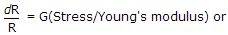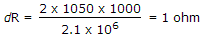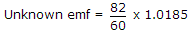Top

# Measurements and Instrumentation

1.

Which of the following voltmeters would you use for measuring voltage across 20 kΩ resistance?

Resistance of voltmeter should be very high as compared to 20 kΩ.

Enter details here

2.

In a resistance strain gauge, G = 2, stress = 1050 kg/cm2, R = 1000 Ω. The value of ΔR will be.

Enter details here

3.

It is required to measure angular position. Out of the transducers

1.Circular potentiometer

2.LVDT

3.E pick up

4.Synchro

The proper devices are

LVDT and E pickup are suitable for linear displacement.

Enter details here

4.

A standard cell of 1.0185 V is used with a slide wire potentiometer. The balance was obtained at 60 cm. When an unknown emf was connected, the balance was obtained at 82 cm. The magnitude of unknown emf is.

Enter details here

5.

To increase Q factor of a coil, the wire should be

Q is high if resistance is low. Therefore, wire should be thick.

Enter details here

6.

A dynamometer wattmeter is connected in ac circuit. The reading will be

Wattmeter measures average power over a cycle.

Enter details here

7.

In which of the transformer is the secondary nearly short circuited under normal operating conditions?

CT is operated with secondary short-circuited otherwise a high emf would be induced across secondary.

Enter details here

8.

Which of the following is true about an impulse voltage?

Impulse is unidirectional (either positive or negative).

Enter details here

9.

The Lissajous pattern observed on screen of CRO is a straight line inclined at 45° to x axis. If X-plate input is 2 sin ωt, the Y-plate input is

When signals to X and Y plates are in phase, the display is a straight line inclined at 45°.

Enter details here

10.

In a ballistic galvanometer, a charge of 100 μC gives a first swing of 25°. The charge required to cause first swing of 50° is